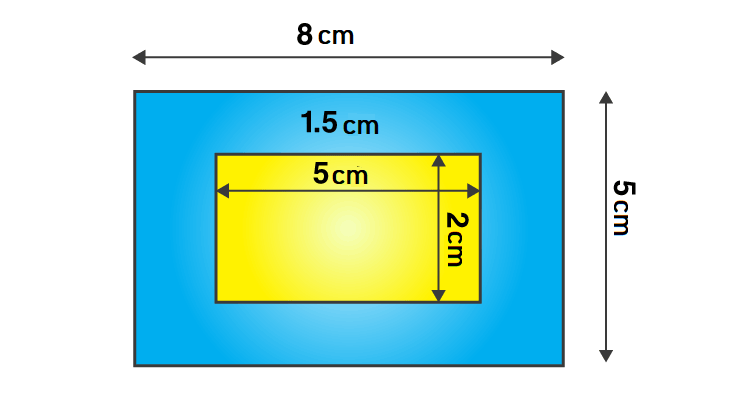# A picture is painted on a cardboard 8 cm long and 5 cm wide such that there is a margin of 1.5 cm along each of its sides. Find the total area of the margin.Given

From the given data we get to know that

Length of the cardboard (L) = 8 cm

Breadth of the cardboard (B) = 5 cm

Find out

We have to determine the total area of the margin.

Solution

Area of the cardboard = length × breadth

= 8 × 5

= 40 cm2

From the figure,

The new length and breadth of the cardboard when the margin is not included are 5 cm and 2 cm.

The new area of the cardboard = 5 × 2

= 10 cm2

The area of margin = Area of the cardboard when the margin is including – Area of the

cardboard when the margin is not including

= 40 – 10

= 30 cm2

Are of the margin=30 cm2(0)(0)